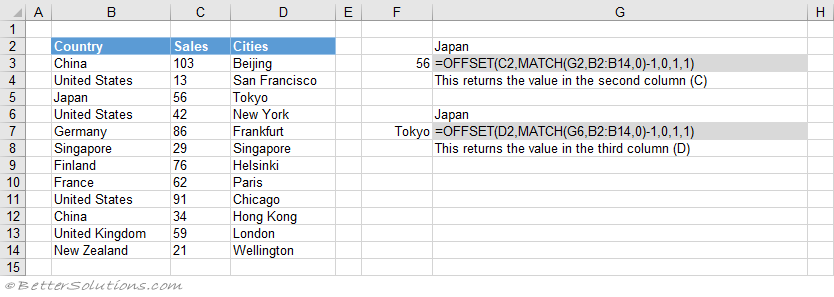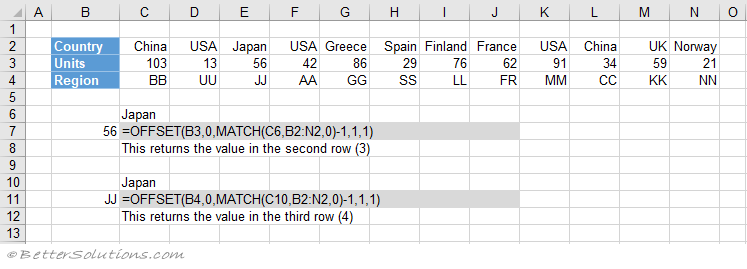# OFFSET and MATCH

Try and avoid using this combination if you can, because OFFSET is a Volatile Function.
Volatile functions recalculate every time any cell changes.
Using the OFFSET function will reduce the performance of your workbook.
You can achieve exactly the same results using the INDEX and MATCH combination.

### Understanding the OFFSET and MATCH Combination

This lets you lookup a value in any column and return a value from any column.
Unlike the VLOOKUP function which can only lookup in the first column.
The MATCH function can be used to find the position of a value in a singe row or column range.
This is used to find the position (or row number) of the value you are looking up in the necessary column.
The OFFSET function can be used to locate the corresponding cell in any column by offsetting from its column heading.

### Return the Exact Match (vlookup equivalent)

An alternative to using the VLOOKUP function is to use the OFFSET function and the MATCH function.### Return the Exact Match (hlookup equivalent)

An alternative to using the HLOOKUP function is to use the OFFSET function and the MATCH function.